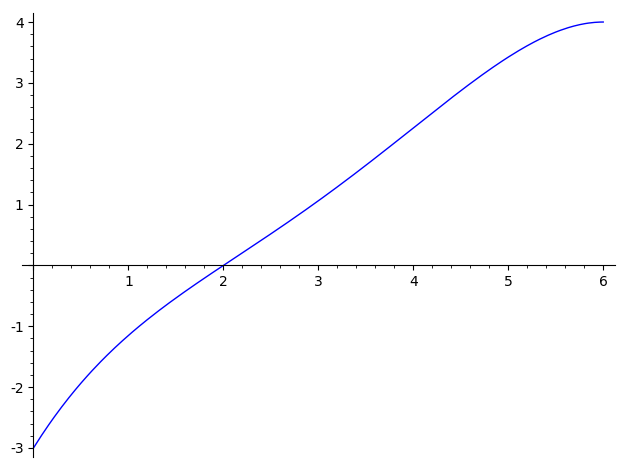# Plot the solution of a system of equations

With

a,b,c,d,e,x = var('a b c d e x')
f(x)=a*x^4+b*x^3+c*x^2+d*x+e
f1(x)=diff(f(x),x)
f2(x)=diff(f(x),x,2)
solve([f(0)==-3, f(2)==0, f2(4)==0, f1(6)==0, f(6)==4], a,b,c,d,e)


I get [[a == (-7/432), b == (43/216), c == (-5/6), d == (5/2), e == -3]]

How can I plot f(x) with these values?

edit retag close merge delete

Sort by » oldest newest most votedsols = solve([f(0)==-3, f(2)==0, f2(4)==0, f1(6)==0, f(6)==4], a,b,c,d,e)
plot(f.subs(sols),(x,0,6))more